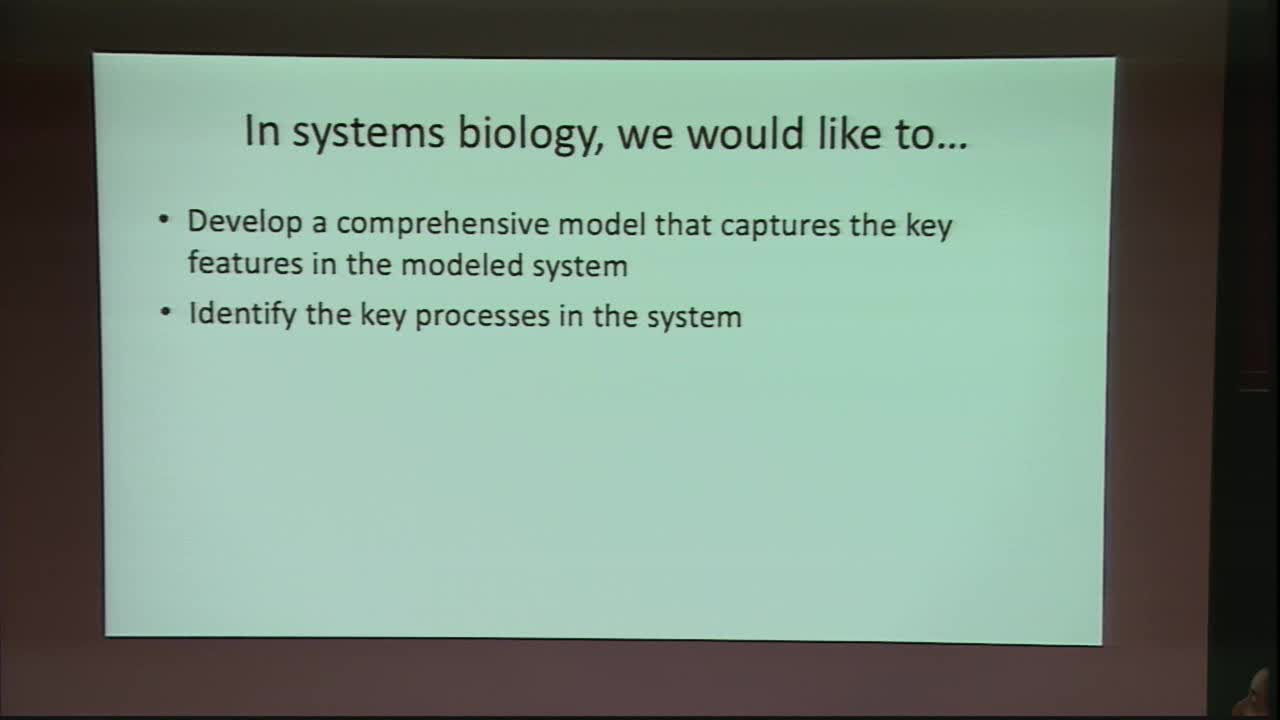## Parameter uncertainty quantification using surrogate models applied to a spatial model of yeast mating polarization

May 29, 2018
IMA
Presenters: Ching-Shan Chou

Watch Video

#### Abstract

Mathematical models in systems biology often have many parameters, such as biochemical reaction rates, whose true values are unknown. When the number of parameters is large, it becomes computationally difficult to analyze their effects and to estimate parameter values from experimental data. This is especially challenging when the model is expensive to evaluate, as is the case for large spatial models. In this work, we introduce a methodology for using surrogate models to drastically reduce the cost of parameter analysis in such models. By using a polynomial approximation to the full mathematical model, parameter sensitivity analysis and parameter estimation can be performed without the need for a large number of model evaluations. We explore the application of this methodology to two models for yeast mating polarization. A simpler non-spatial model is used to demonstrate the techniques and compare with published results, and a large spatial model is used to demonstrate the computational savings afforded by this method.